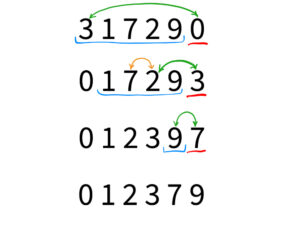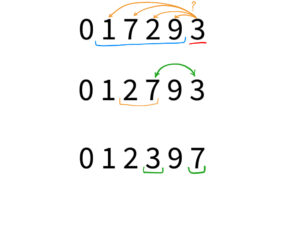# Quick Sort algorithm in C#### Quick sort algorithm in C#

Quick Sort is a widely used sorting algorithm that is based on the divide-and-conquer approach. It is considered one of the most efficient sorting algorithms with an average time complexity of O(n log n). The basic idea behind quick sort is to partition the array into two sub-arrays, one containing elements less than a pivot element and the other containing elements greater than the pivot element. The pivot element is chosen in such a way that it divides the array into two roughly equal-sized partitions. This process is then repeated recursively on the two sub-arrays until the entire array is sorted. Quick sort algorithm in C# is represented as a built-in method, however, you can rewrite it on your own.

In C#, the built-in Array.Sort() method and the List<T>.Sort() method use a variant of the QuickSort algorithm to sort the elements of the array or list. However, sometimes you may need to implement your own custom QuickSort algorithm to suit your specific needs. For example, if you have a custom type of object that doesn’t implement the IComparable interface, you can’t use the built-in sorting methods.

FLASH SALES
\$29.95
NEW
\$249.00
Become a C# PRO and land your first developer job!

### Custom version of Quick sort

Here is an example of how to implement a custom quick sort algorithm in C#:

This code is an implementation of the Quick Sort algorithm, which is a divide-and-conquer algorithm used to sort an array of integers. The Sort() method is a public static method that takes in an integer array, arr, and two integers, left and right, as arguments. The method first checks if the left index is less than the right index. If it is, the method calls the private static Partition() method and assigns the returned value to a variable named pivot.

The Partition() method takes in the same three arguments as the Sort() method and is used to partition the array and select a pivot element. The pivot element is chosen to be the last element of the array. The method initializes a variable named i to be left – 1 and a variable named j to be left. It then enters a for loop that iterates from the left index to the right index (not including the right index). Inside the for loop, the method checks if the current element is less than or equal to the pivot element. If it is, the method increments i and swaps the current element with the element at the i index.

After the for loop, the method swaps the element at the i+1 index with the pivot element, and return i+1 as the pivot index.

Finally, back in the Sort() method, the method is called recursively to sort the left and right partitions of the array, taking the pivot-1 index and pivot+1 index as the new right and left bounds, respectively. This process continues until the left index is no longer less than the right index, at which point the array is fully sorted.

This implementation of the quick sort algorithm is for an array of integers, but the same logic can be applied to arrays of other data types or even to custom types of objects by making a few modifications. For example, you can pass a custom comparison delegate or a custom comparer object to the Sort and Partition methods to define the sorting logic.

### Visualization

Let’s imagine that we want to sort an array with the Quick sort and will go along with our algo.

As you know, the actual sorting happening in our Partition method. So let’s see what happens on each level of recursion. You can see them in the picture below:The value underlined in red color is our pivot. On the first level, it is 0 on the second, 3, and on the third, it is 7.

The blue line highlights the unsorted part of our array. And arrows represent changes. The orange one shows changes made by the first swap, and the green one by the second swap.

Now that you know the rules, you see that on the first line, our pivot is the smallest value. And the only action we did was the second swap, which, actually, just put our pivot between values that are smaller and bigger than it is. Moreover, as you see, we do it on each recursion level.

The next line shows us two types of swaps. Because the pivot is three and it is one of the best possible pivots in this case, as there are smaller and bigger values. And as you see, here we have done the biggest part of our sorting process. So I would like you to take a close look at this level in the picture below:This picture schematically shows us the for loop from the Partition method and the changes that have been made. First, we were looking for the best place for our pivot. And, at the same time, we were sorting the left side of our array. And in the end, we just placed the pivot where it should be.

### Summary

In conclusion, quick sort is an efficient sorting algorithm that can be effectively implemented in C#. The built-in Array.Sort() method and the List<T>.Sort() method use a variant of the QuickSort algorithm to sort the elements of the array or list, but sometimes you may need to implement your own custom quick-sort algorithm to suit your needs.